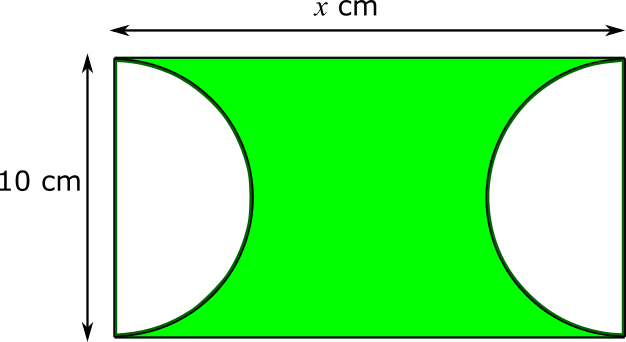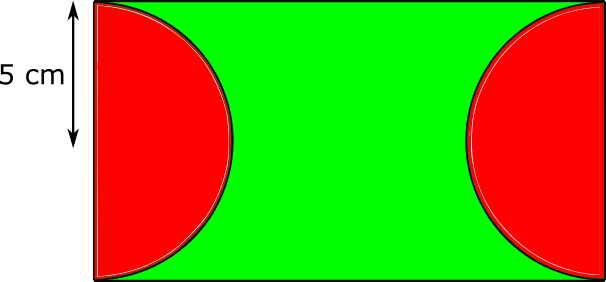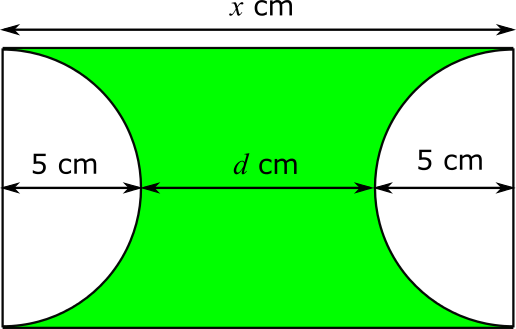#### You may also like### Some(?) of the Parts

A circle touches the lines OA, OB and AB where OA and OB are perpendicular. Show that the diameter of the circle is equal to the perimeter of the triangleA 1 metre cube has one face on the ground and one face against a wall. A 4 metre ladder leans against the wall and just touches the cube. How high is the top of the ladder above the ground?Four rods are hinged at their ends to form a convex quadrilateral. Investigate the different shapes that the quadrilateral can take. Be patient this problem may be slow to load.

# Semicircle Distance

##### Age 14 to 16 ShortChallenge Level

Let the length of the rectangle be $x$. Then by considering the area of the whole rectangle, we can find $x$.

The area of the whole rectangle can be found using length $\times$ width = $10x$, as shown below.This must be equal to the shaded area added to the total area of the two semicircles. The radius of each semicircle must be half of 10 cm, which is 5 cm.So the red area is equal to $\pi\times 5^2$ cm$^2=25\pi$ cm$^2$, because the two red semicircles could be stuck together to make a circle of radius 5 cm.

That means that the total area of the rectangle must be $125 + 25\pi$ cm$^2$, so $10x=125+25\pi$, so $x=\dfrac{125+25\pi}{10}=12.5+2.5\pi$.

Now, as shown below, the shortest distance can be found using $x$.$d=x-5-5=x-10$, so $d=12.5+2.5\pi-10=2.5+2.5\pi$ cm.

You can find more short problems, arranged by curriculum topic, in our short problems collection.